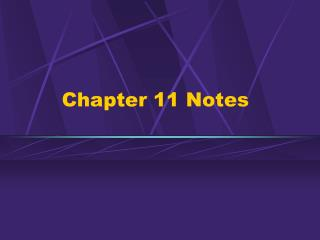# Chapter 11 Notes - PowerPoint PPT PresentationDownload PresentationChapter 11 Notes

Chapter 11 Notes
Download Presentation## Chapter 11 Notes

- - - - - - - - - - - - - - - - - - - - - - - - - - - E N D - - - - - - - - - - - - - - - - - - - - - - - - - - -
##### Presentation Transcript

1. Chapter 11 Notes

2. Section 1 - Pressure • Pressure - equal to the force exerted on a surface divided by the total area over which the force is exerted. • Pressure = force/area • pressure units = pascal • increase surface area, decrease pressure • ice skates • fluid - substance which can easily flow Chapter 11

3. All of the forces exerted by the individual molecules in a fluid add together to make up the pressure exerted by the fluid. • Balanced pressure - air pressure • pressure at a given level in a fluid is the same in all directions • air pressure decreases as elevation increases • water pressure increases as depth increases Chapter 11

4. Section 2 - Transmitting Pressure in a Fluid • Pascal’s principle - When force is applied to a confined fluid, an increase in pressure is transmitted equally to all parts of the fluid. Chapter 11

5. Hydraulic System • Multiplies a force by applying the force to a small surface area. • The increase in pressure is then transmitted to another part of the confined fluid, which pushes on a larger surface area. • Small force over small area results in a larger force exerted by a larger area at another place. • Car brakes, repair shop lift, fire truck ladder Chapter 11

6. Section 3 - Floating and Sinking • Buoyant force - acts in an upward direction, against the force of gravity. • Makes an object feel lighter • Archimedes’ principle - the buoyant force on an object is equal to the weight of the fluid displaced by the object • floating and sinking - pg. 362 Chapter 11

7. density = mass/volume units g/mL or g/cm3 • water density = 1 g/mL pg.363 Chapter 11

8. Section 4 - Applying Bernoulli’s Principle • Wright brothers Chapter 11

9. Bernoulli’s principle • The pressure exerted by a moving stream of fluid is less than the pressure of the surrounding fluid. • Air moves at different speeds above and below objects. • Difference in pressure causes lift or downward force • wings pg. 367 Chapter 11

10. Chapter 11 - Vocabulary List • Pressure • Pascal’s principle • hydraulic system • buoyant force • Archimedes’ principle • Bernoulli’s principle Chapter 11